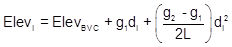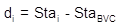### 3. Curve Equation

A constant grade change rate means the curve does not have a fixed radius - it's continually changing. This provides a smoother transition when vertical travel direction is changed. A circular arc isn't generally used as a vertical curve because it has constant curvature with a fixed radius. While acceptable at lower speeds, at higher speeds there is a tendency for a vehicle to "fly" at the highest point of a crest curve or "bottom out" at the lowest point of a sag curve. While desirable for roller coaster design, upsetting passenger stomachs is generally frowned upon in road design.

Instead, a parabolic arc is used. A parabola tends to flatten at the vertical direction change making for a more comfortable transition.

The general equation for a parabolic curve, Figure B-7, is Equation B-6.Equation B-6
Figure B-7
Parabola

The equation consists of two parts, Figure B-8:

• a straight line, bx+c, which is tangent to the curve at its beginning (b is the slope, c is the y intercept), and,
• an offset, ax2, which is the vertical distance from the tangent to the curve.Figure B-8 Tangent and Offset

Figure B-9 shows the parabolic arc in terms of vertical curve nomenclature.Figure B-9 Curve Terms

The curve begins at the BVC station and elevation.
The tangent line slope is g1, the incoming grade.

At distance d:

• the tangent elevation is g1d+ElevBVC
• the tangent offset is ad2, where a is a function of k

The parabolic equation written in curve terms is the Curve Equation, Equation B-7.Equation B-7

(For a complete graphic derivation of the Curve Equation see the Family of Curves section.)

di is the distance from the BVC to any point i on the curve. It is computed from Equation B-8 and ranges from 0 to L.Equation B-8

Recall the previous warning on grade and distance units. In Equation B-5 the combination should be either grades in percent with distances in stations, or grades as a ratio with distances in feet. Remember that curve length, L, is a distance and must play by the same rules.

The grade at any point on the curve is a function of the beginning grade along with k and the distance from the BVC, Equation B-9.Equation B-9

Using Equations B-7 through B-9 allows us to compute the elevation and grade at any point along the curve.  To compute the elevation of the curve's midpoint, L/2 would be used for di in Equation B-7.

A geometric characteristic of a parabolic vertical curve is at its midpoint the vertical offsets from the PVI to the curve and from the curve to the chord, o in Figure B-10, are the same.Figure B-10 Midcurve Offsets

Besides using Equation B-7, another way to compute the midpoint elevation is from the following:

• The chord's midpoint elevation is the average elevation of the BVC and EVC.
• The curve's midpoint elevation is the average elevation of the chord midpoint and PVI.

The curve midpoint generally isn't any more or less important than other curve points. Being able to compute its elevation two ways, however, can be used as a partial math check